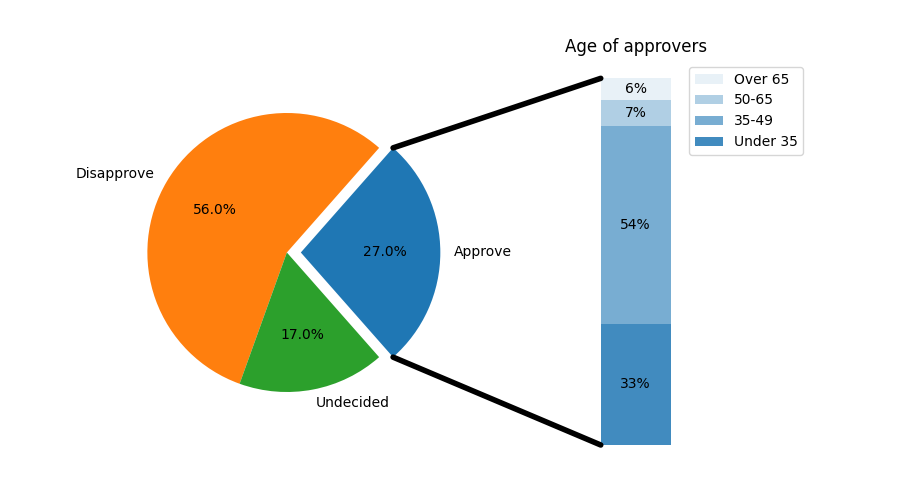# Bar of pie#

Make a "bar of pie" chart where the first slice of the pie is "exploded" into a bar chart with a further breakdown of said slice's characteristics. The example demonstrates using a figure with multiple sets of axes and using the axes patches list to add two ConnectionPatches to link the subplot charts.

import matplotlib.pyplot as plt
from matplotlib.patches import ConnectionPatch
import numpy as np

# make figure and assign axis objects
fig, (ax1, ax2) = plt.subplots(1, 2, figsize=(9, 5))

# pie chart parameters
overall_ratios = [.27, .56, .17]
labels = ['Approve', 'Disapprove', 'Undecided']
explode = [0.1, 0, 0]
# rotate so that first wedge is split by the x-axis
angle = -180 * overall_ratios
wedges, *_ = ax1.pie(overall_ratios, autopct='%1.1f%%', startangle=angle,
labels=labels, explode=explode)

# bar chart parameters
age_ratios = [.33, .54, .07, .06]
age_labels = ['Under 35', '35-49', '50-65', 'Over 65']
bottom = 1
width = .2

# Adding from the top matches the legend.
for j, (height, label) in enumerate(reversed([*zip(age_ratios, age_labels)])):
bottom -= height
bc = ax2.bar(0, height, width, bottom=bottom, color='C0', label=label,
alpha=0.1 + 0.25 * j)
ax2.bar_label(bc, labels=[f"{height:.0%}"], label_type='center')

ax2.set_title('Age of approvers')
ax2.legend()
ax2.axis('off')
ax2.set_xlim(- 2.5 * width, 2.5 * width)

# use ConnectionPatch to draw lines between the two plots
theta1, theta2 = wedges.theta1, wedges.theta2
center, r = wedges.center, wedges.r
bar_height = sum(age_ratios)

# draw top connecting line
x = r * np.cos(np.pi / 180 * theta2) + center
y = r * np.sin(np.pi / 180 * theta2) + center
con = ConnectionPatch(xyA=(-width / 2, bar_height), coordsA=ax2.transData,
xyB=(x, y), coordsB=ax1.transData)
con.set_color([0, 0, 0])
con.set_linewidth(4)

# draw bottom connecting line
x = r * np.cos(np.pi / 180 * theta1) + center
y = r * np.sin(np.pi / 180 * theta1) + center
con = ConnectionPatch(xyA=(-width / 2, 0), coordsA=ax2.transData,
xyB=(x, y), coordsB=ax1.transData)
con.set_color([0, 0, 0])